# Electronics I for ECE

## Decibels and Power Ratios

Sound power ratios are sensed in human hearing as ratios of power.  An increase in sound power by a factor of 10 sounds like the same difference whether it is a change from a whisper or a change from a roar.  Brightness is also sensed logarithmically.  Numbers that change in the same way are call logarithms.

To explain logarithms and how they are used in decibel notation, we begin with a graph of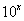.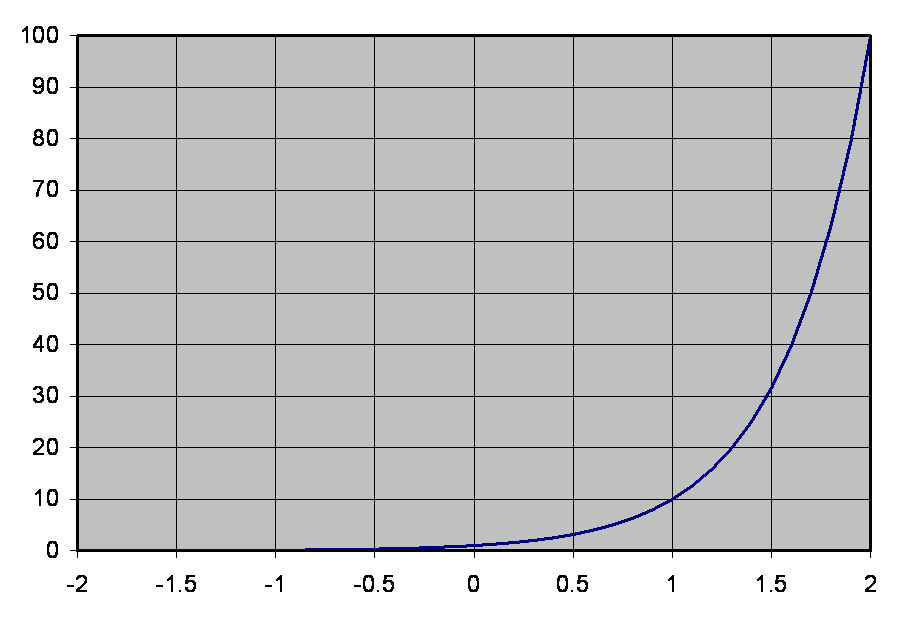Figure 1.  Graph of 10x versus x

Note that as x changes by 1, the value of 10x changes by a factor of 10.  The inverse of this relationship is the logarithm to the base 10, or log10(y).  The logarithm to the base 10 is graphed below.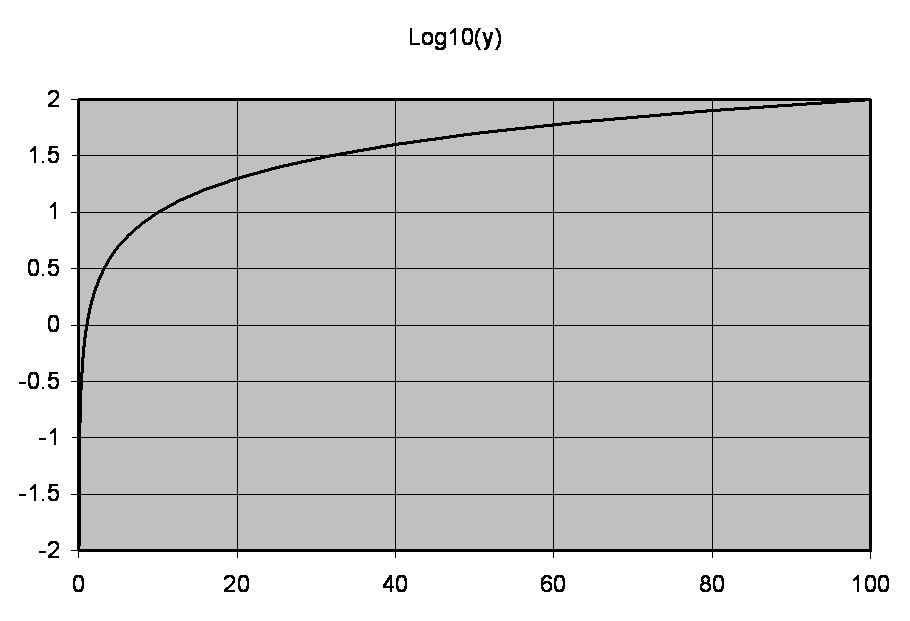Figure 2.  Log10(y) versus y

The curves in the two plots are the same, with the x and y axes interchanged.  Note that as y increases or decreases by a factor of 10, log10(y) increases or decreases by 1.

The logarithm was used by Alexander Graham Bell to define power ratios as a unit, which was later called a Bell.  A tenth of a Bell is a decibel, or dB, and the B is capitalized because it stands for AGB's last name.  Decibels are always defined in terms of power ratios:.

A dB difference between two powers that is positive means that the second power is higher than the first.  Conversely, if a dB figure is negative, it means that there is a decrease in power.

## Decibel Specifications of Powers Relative to an Impedance

Often we measure or specify power in a 75-Oohm cable, 600-Ohm transmission line, or some similar situation where we have a single power.  We reference that power to either 1 Watt or one milliwatt, usually a milliwatt.  When we specify powers without an impedance, the equation is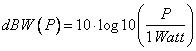for power relative to a Watt, or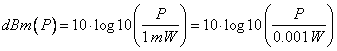.

The dBW and dBm powers will differ by 20 dB (why?).

When we specify an impedance, we can measure the voltage and find the power by Ohm's Law,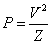where V is the amplitude in Volts (RMS voltage for a sine wave, noise, or other complex signal) and Z is the impedance in ohms.  Note that the logarithm has the property that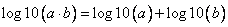so that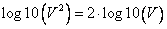.

We can use Ohm's Law to write the dB difference between to signals in terms of their voltages at the same impedance as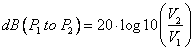.

The text gives voltages for 1 milliwatt at 600 Ohms as 0.775 Volts, and at 75 Ohms as 0.274 Volts.

## Voltages for dBm(Z), where Z is an Impedance

Occasionally voltages for 1 milliwatt at impedances of 50 Ohms, 75 Ohms, 600 Ohms, etc. are specified or required.  The simplest way to measure power levels in dBm for a specific impedance is to find the peak or RMS voltage for 1 mW at that impedance and then measure the peak or RMS voltage of the signal.  In general, this can be done for any given impedance as follows.

1. Use Ohm's Law to find the RMS voltage for a 1 milliwatt signal as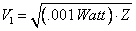where Z is the impedance in Ohms – which can be 50 Ohms, 75 Ohms, 600 Ohms, 50 Ohms, 300 Ohms, or whatever.
2. Measure the voltage of the signal on the terminal or line of Z Ohms impedance.
3. Find the signal power in dBm(Z) as.

## Transfer Functions of Simple R-C Circuits

We write the complex form of the current and voltage by using an appended "z" in the variable name, we can write the voltage and current law using a modified version of Ohm's Law.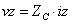where the quantity that takes the place of resistance,is the impedance of the capacitor,.

Examine the circuit of Figure 3 below.  When the input voltage is a sine wave, the impedance concept allows the voltage divider equations to be used with the impedance concept to provide the amplitude of the output and its phase relative to the input.Figure 3 Simple Low-Pass RC Circuit with Source Voltage

We see that this is a simple voltage divider, and we can write the output as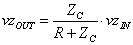.

We note that the ratio of the output and the input is.

We call this ratio the transfer function of the circuit and denote it by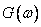where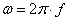is called the angular frequency.  The transfer function has the property of giving the steady-state phase and amplitude of the output of the circuit, relative to a constant amplitude and phase sine wave input, as a function of the circuit values and the frequency of the sine wave.   The amplitude and phase plots are shown below with a logarithmic frequency scale for a resistance of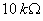and a capacitance of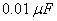.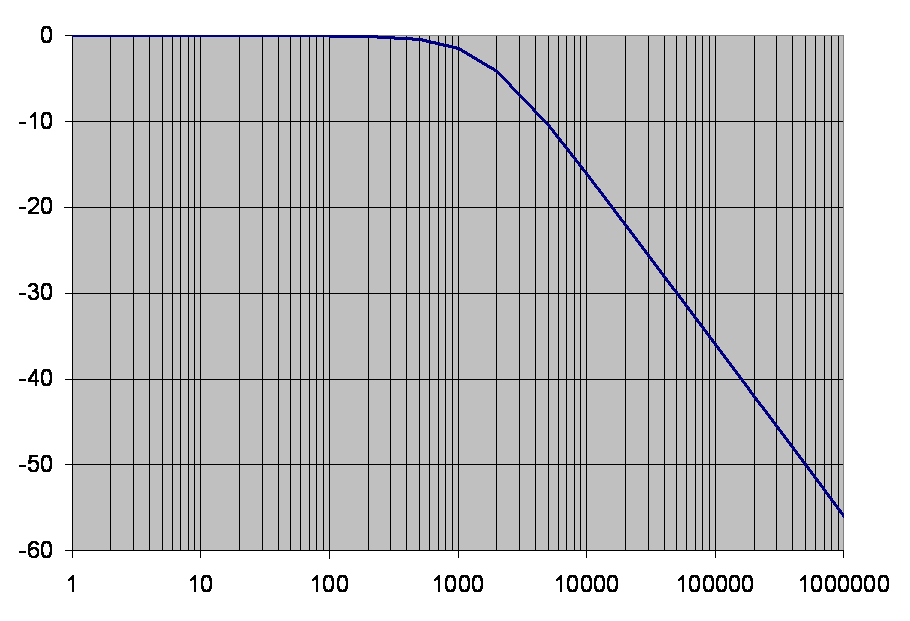Figure 4.  Amplitude Response of Low-Pass RC CircuitFigure 5.  Phase Response of Low-Pass RC Circuit

## The Corner or Roll-Off Frequency

Note in Figure 4 above that the transfer function is 3 dB down at a frequency of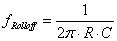.

For a resistance ofand a capacitance of, this frequency is 1591.55 Hz.  This can be predicted from the equation for the transfer function G(f) because its value is 1/(1 + j) at that frequency.  Note that the phase lag of the output relative to the input is 45 degrees, as shown in Figure 5 above.

For frequencies well below the rolloff frequency,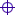The amplitude of the transfer function is about unity.The phase lag of the transfer function is small.

For frequencies well above the rolloff frequency,The amplitude of the transfer function rolls off at 20 dB per decade, or 6 dB per octave.The plot of the amplitude of the transfer function can be approximated by its asymptote, which is a straight line intersecting 1.0 at the rolloff frequency, and with a slope of -20 dB per decade.The phase lag of the transfer function is about 90 degrees.